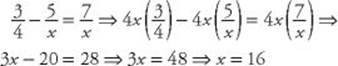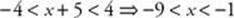﻿ ﻿ABSOLUTE VALUE, RADICAL, AND FRACTIONAL EQUATIONS AND INEQUALITIES - Equations and Inequalities - ALGEBRA - SAT SUBJECT TEST MATH LEVEL 1

## ALGEBRA## CHAPTER 6Equations and Inequalities### ABSOLUTE VALUE, RADICAL, AND FRACTIONAL EQUATIONS AND INEQUALITIES

The six-step method works in other situations as well. The next four examples illustrate the proper use of this method in equations and inequalities in which the variables are in denominators, between absolute value bars, or under radical signs.

EXAMPLE 5: For what value of x isA second, and in this case slightly easier, solution to Example 5 is to start by addingto each side:Therefore, 3x = 48 and x = 16

EXAMPLE 6: For what values of x is?

As in the second solution to Example 5:Now be careful. Cross multiplying the inequalityis essentially multiplying each side of the inequality by 4x.

So now you must consider two cases. If x is positive, so is 4x, and multiplying by 4x will preserve the inequality. However, if x is negative, so is 4x, and multiplying by 4x will reverse the inequality. Therefore, you must treat the two cases separately.

Case I: x > 0.So the positive solutions to the inequality are all numbers greater than 16.

Case II: x < 0.So the negative solutions to the inequality are all negative numbers that are less than 16. Since every negative number is less than 16, every negative number is a solution.

Finally, by combining the two cases, we see that the solution set is {x | x < 0 or x > 16}.

EXAMPLE 7: For what values of x is 3|x + 5| – 5 = 7?EXAMPLE 8: For what values of x is 3|x + 5| – 5 < 7?Now recall from KEY FACT A2 that this means:If you have to solve an equation such as, which involves a radical, 4x use the six-step method, treating the radical as your variable. After step 6, when you have a radical equal to a number, perform one final step: raise each side to the same power. For example, if the radical is a square root, square both sides; if the radical is a cube root, cube both sides.

EXAMPLE 9:EXAMPLE 10:EXAMPLE 11:Sincecannot be negative, no real number x satisfies the equation.

TIPIf you are solving an equation that has a square root or any even root equal to a negative number, the equation has no real solutions.

﻿